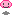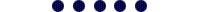Neither one nor Many

October 19 2012

## Multiple iterators that use the same begin() or end() functions.

In C++ you cannot differentiate based on the type-to-return. Like have two begin() methods in a class that return different iterators.

``````class foo
{
public:
some_iterator begin()
{
return some_iterator();
}

// Not possible
other_iterator begin()
{
return other_iterator();
}
};

some_iterator it = fooinstance.begin();
``````

There is also no template syntax to implement a begin() method for this purpose. Note that you cannot use "straightforward" polymorphism because the subclasses are on the LHS of the assignment.

## I still wanted it though and came up with the following solution.For example an instance of a NumberRange class provides two iterators the default "iterator" simply outputs all the numbers. The "cumulative_iterator" outputs all numbers cumulatively.

``````int main(int argc, char **argv)
{
NumberRange range(1, 10);

cout << "NumberRange::iterator:" << endl;
for (NumberRange::iterator iter = range.begin(); iter != range.end(); iter++)
cout << *iter << endl;

cout << "NumberRange::cumulative_iterator:" << endl;
for (NumberRange::cumulative_iterator iter = range.begin(); iter != range.end(); iter++)
cout << *iter << endl;

return EXIT_SUCCESS;
}

/**
* Desired output:
* -----------------------------------------------------------
* ksh\$ g++ iterators.cpp &&./a.out
* Constructing NumberRange object with numbers 1 to 10
* NumberRange::iterator:
* 1
* 2
* 3
* 4
* 5
* 6
* 7
* 8
* 9
* 10
* NumberRange::cumulative_iterator:
* 1
* 3
* 6
* 10
* 15
* 21
* 28
* 36
* 45
* 55
*/
``````

I really like this as an API because if you want to change the way of iterating through the range (in this example), you only change NumberRange::iterator to something else.

## Implementation of NumberRange

``````class NumberRange
{
public:
NumberRange(int rangebegin, int rangeend)
{
cout << "Constructing NumberRange object with numbers " << rangebegin << " to " << rangeend << endl;

for (int i=rangebegin; i<=rangeend; i++)
numbers_.push_back(i);
}

NumberIter<void> begin()
{
return NumberIter<void>(numbers_, 0);
}

NumberIter<void> end()
{
return NumberIter<void>(numbers_, numbers_.size());
}

typedef NumberIter<Traits_Normal> iterator;
typedef NumberIter<Traits_Cumulative> cumulative_iterator;

private:
vector<int> numbers_;
};
``````

It's a very simple implementation. It stores the numbers in a vector. The functions begin() and end() provide iterators of NumberIter. NumberIter is a templated class with traits. Possible traits that we are going to define are: void, Traits_Normal, Traits_Cumulative. I prefer to use void where the specific Trait is not yet known. I could have also have made a Traits_Null.

NumberRange only works with NumberIter<void> because begin() does not know what specifc NumberIter instance to return (Traits_Normal or Traits_Cumulative). In the assignment "NumberRange::iterator iter = range.begin()" the NumberIter<void> is converted into a NumberIter<Traits_Normal>. (NumberRange::iterator is a typedef for NumberIter<Traits_Normal>.)

## Implementation of NumberIter

This class is templated to provide multiple kinds of iterators, by using NumberIterTraits. These traits provide the implementation of the specific iterators. So this class only provides the API.

``````template <typename T, typename Traits = NumberIterTraits<T> >
class NumberIter: public std::iterator< std::forward_iterator_tag, string >
{
public:

// Constructors
NumberIter(const vector<int> &numbers, size_t seq)
: sequence_(seq), numbers_(numbers)
{}

// Copy constructor
NumberIter(const NumberIter<void> &other)
{
numbers_ = other.numbers_;
sequence_ = other.sequence_;
}

// Operators
const int operator*() const
{
return Traits::next(numbers_, sequence_);
}

NumberIter & operator++(int)
{
sequence_++;
return *this;
}

template <typename N>
bool operator==(const NumberIter<N>& other)
{
return sequence_ == other.sequence_;
}

template <typename X>
bool operator!=(const NumberIter<X>& other)
{
return !((*this) == other);
}

private:

vector<int> numbers_;
size_t sequence_;

friend class NumberIter<Traits_Normal>;
friend class NumberIter<Traits_Cumulative>;
};

``````
• Constructor takes a copy of the numbers vector, which is really inefficient. But I wanted to keep the example simple. Sequence parameter is the current position of the iterator.
• There is a copy constructor (used in the assignment "NumberRange::iterator iter = range.begin();")
• operator* returns the current value of the operator. Note that the traits implement different processing and return.
• operator++ increments the sequence.
• operator== and operator!= are required for "i != range.end()".

## Traits classes

``````template<typename T> class NumberIterTraits;
template<> class NumberIterTraits<void>
{
public:
static int next(const vector<int> &numbers, size_t sequence)
{
throw logic_error("NumberIterTraits<void>::next should not be used.");
}
};

class Traits_Normal;
template<> class NumberIterTraits<Traits_Normal>
{
public:
static int next(const vector<int> &numbers, size_t sequence)
{
return numbers[sequence];
}
};

class Traits_Cumulative;
template<> class NumberIterTraits<Traits_Cumulative>
{
public:
static int next(const vector<int> &numbers, size_t sequence)
{
if (sequence < 0)
return 0;

int value = 0;

for (int i=0; i <= sequence; i++)
value += numbers[i];

return value;
}
};
``````

The Traits_Normal version simply returns the number at the index. The Traits_Cumulative sums all numbers from first to current index.

Note that to add another iterator you only need to add another Traits class. (Well in my case another typedef in NumberRange for consistency as well. But you could do without and omit them like "for (NumberIter<Traits_Something> i = range.begin(); ...)".)

[Edit: also a friend class declaration in NumberIter. That's so that the "generated" NumberIter classes can reference internals. Personal preference over adding more class functions.]

[Edit2: You could add a Traits_Reverse with "return numbers[numbers.size() - ++sequence];"]

## Final notes

IIRC there are some compilers that require an implementation of "operator=" for the conversion in "NumberRange::iterator = range.begin()". They refuse to use the copy constructor for this statement. In that case use this on the class.

``````NumberIter operator=(NumberIter<void> val)
{
numbers_ = val.numbers_;
sequence_ = val.sequence_;
return *this;
}
``````

Complete source code can be downloaded here.There are probably more alternatives for this, i.e. you could probably do without templates.

The iterators in this example are not fully std compliant. I.e. you cannot use them in functions from #include <algorithm>.

Code tested on gcc version 4.3.4 [gcc-4_3-branch revision 152973] (SUSE Linux).Comment may not be visible immediately, because I process everything manually.**

**) I plan to automate this.., but it's on my ToDo since for ever..

Author:Ray Burgemeestre
february 23th, 1984

Topics:
C++, Linux, Webdev

Other interests:
Music, Art, Zen